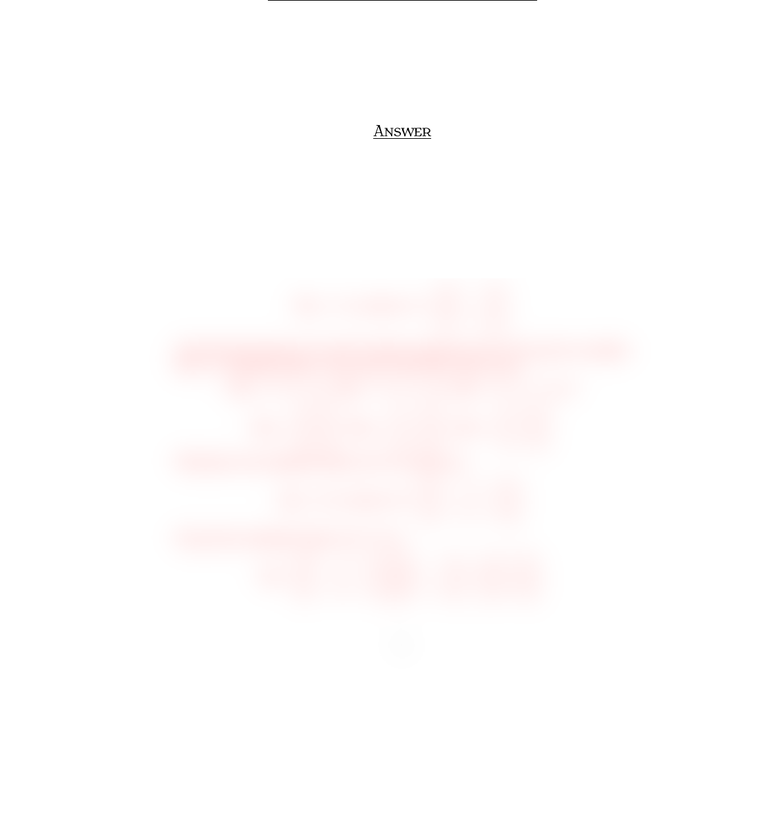# Quiz6mt210Sample1Ans

16 views1 pagesMT Q  S 
İ S. Y
M , 
Surname, Name:
Q . §. M O
Let T:R2ÏR3be the linear transformation deﬁned by
(x1, x2)(x1+x2, x2, x1x2)
and S:R3ÏR3be the linear transformation deﬁned by
(x1, x2, x3)(x1+x2+x3, x1x2x3,x1x2x3).
Find the standard matrix of ST.
A
Note that the domain of Tis R2and the codomain is R3. We need to consider
the 2×2identity matrix. We need to ﬁnd T(e1), T(e2):
T(e1) = (1,0,1), T(e2) = (1,1,1) or
T(e1) =
1
0
1
, T(e2) =
1
1
1
.
Therefore, the standard matrix Aof Tis given as
T(x) = A·xwhere A =
1 1
0 1
11
.
Note that the domain of Sis R3and the codomain is R3. We need to consider
the 3×3identity matrix. We need to ﬁnd S(e1), S(e2), S(e3):
S(e1) = (1,1,1), S(e2) = (1,1,1), S(e3) = (1,1,1), or
S(e1) =
1
1
1
, S(e2) =
1
1
1
, S(e3) =
1
1
1
.
Therefore, the standard matrix Bof Sis given as
S(x) = B·xwhere B =
1 1 1
111
111
.
We get the standard matrix of STas
BA =
1 1 1
111
111
1 1
0 1
11
=
0 2
0 2
0 2
.
1
Unlock document

This preview shows half of the first page of the document.
Unlock all 1 pages and 3 million more documents.